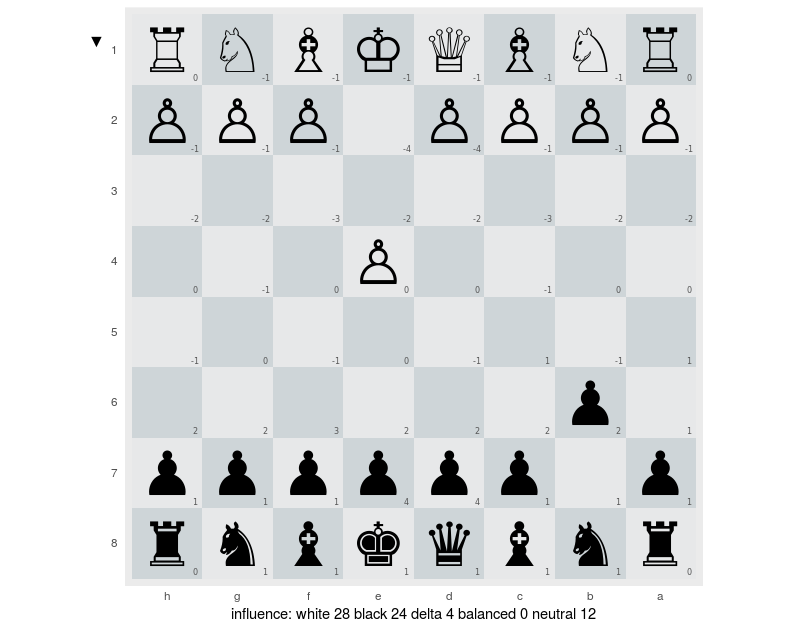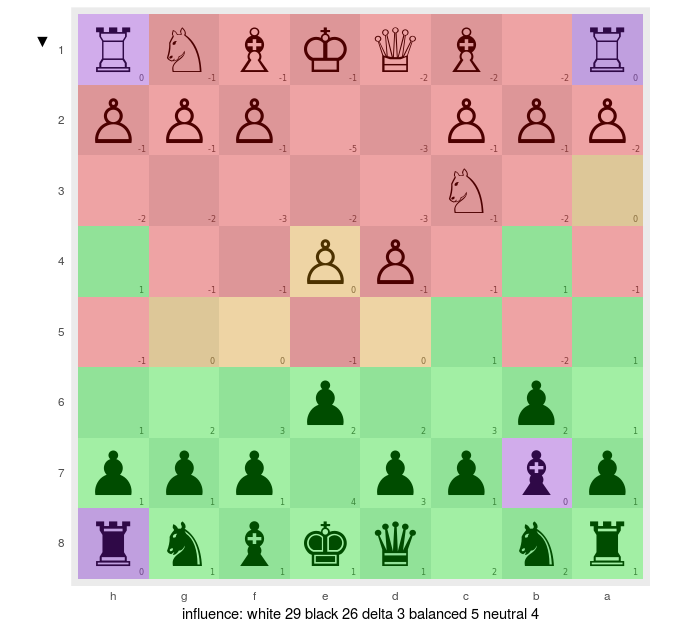by Neonira

• games
• EN

• chess
• computer
• R

### Experimenting chessboard influence

This is the third post of a serie, dedicated to chessplay and more specifically to the battle of influence on the chessboard.
We are now trying to compute influence zone over the chessboard.

In cas you missed previous posts, you may read part 1 and part 2.

### Elaborating influence computation

We have seen that using rchess, we can get easily the full list of moves played during a chess game, and at anytime, we can retrieve the FEN string and compute position image for each move.
Now, we need to proceed to position analysis in order to achieve following results

• compute each board squarre influence for white and black sides
• generate chessboard images to expose those results
• generate some diagrams to understand how it flows thru the whole chess game
• proceed to a video montage to understand if knowing/tracking the chessboard influence could help us improve our play.

### Chess board influence computation

Trying to determine if a chess squarre is under white side control or black side control, requires many different computations, according to the side, the piece and the current position being studied. Moreover, a binary classification result is wrong here, as a squarre could be under control, out of control, under balanced control or, not even disputed.

#### Piece influence computation

As each piece has its owns incidence, we’ll stick to an object oriented approach to solve the computation. We will create a base class named AbstractPiece and derived it for each kind of piece, Pawn, Knight, Bishop, Rook, Queen, and King.
I’ll use R6 package to do so. Here is the code for the base class

``````AbstractPiece <- R6Class('AbstractPiece',
portable = TRUE,
public = list(

initialize = function(nam_s, san2_s) {
sc <- cleanString(nam_s)
if (missing(nam_s) | nchar(sc) == 0) stop('piece name must be provided')
private\$nam <- sc
private\$side <- tolower(nam_s) != nam_s# true for white, false for black
if (nchar(san2_s) != 2) stop(paste0(san2_s, 'must be 2 character long'))
private\$col <- which(letters == substring(san2_s, 1, 1))
private\$row <- as.integer(substring(san2_s, 2, 2))
private\$figurine <- list(
'p' = '♟',
'n' = '♞',
'b' = '♝',
'r' = '♜',
'q' = '♛',
'k' = '♚',
'P' = '♙',
'N' = '♘',
'B' = '♗',
'R' = '♖',
'Q' = '♕',
'K' = '♔'
)
},

# allows to get the consumption and units to bill
# @param data_dt, a data table
# @return a vector of 64 integers with 0 on non controlled squarres, 1 on controlled ones
# @exception might not throw exception except the base abtract instance, if any
getControlledSquarres = function(data_dt) {
stop(paste('AbstractPiece::getControlledSquarres', private\$abstractMsg))
},

# allows to retrieve easily service name
# @param NA
# @return piece name
# @exception NA
getName = function() { private\$nam },

getRow = function() { private\$row },

getCol = function() { private\$col },

getSide = function() { private\$side },

getVerbosity = function() { private\$verbose },

setVerbosity = function(verbose_b) { private\$verbose = verbose_b; },

getVector = function() { vector(mode = "integer", length = 64) },

getSan = function() { paste0(letters[private\$col], private\$row) },

getSignature = function() { paste0(private\$figurine[[private\$nam]], self\$getSan()) },

getFigurine = function(keyletter_s = NA) {
kl <- if (is.na(keyletter_s)) private\$name else keyletter_s
private\$figurine[[kl]]
},

numToSan = function(i) {
stopifnot(i >= 1 & i <= 64)
co <- i %% 8
if (co == 0) co <- 8
ro <- ceiling(i / 8)
paste0(letters[co], ro)
},

print = function(...) {
cat('class=', paste0(class(self), collapse=','), ' @=', address(self), '\n', sep='')
cat('name: [', private\$nam,'] ', letters[private\$col], private\$row, '\n', sep='')
if (!missing(...)) {
y <- as.vector(...)
catn(strJoin(sort(sapply(which(y > 0), self\$numToSan))))
m <- t(matrix(..., nrow = 8, ncol = 8, dimnames = list(letters[1:8], 1:8)))
if (!private\$side)
print(m)
else
print(apply(m, 2, rev))
}
}

),
private = list(nam = NA,
col = NA,
row = NA,
side = NA,
figurine = NA,
verbose = FALSE,
abstractMsg = 'this method should never be called')
)

``````
##### Remarks

R6 code shown here might be sub-optimal. I had to find my way through several constraints emaning from R object oriented design.

Main issues I faced are

• declaring a pure abstract class. I relied on a constructed approach, as I haven’t found how to do that, purely relying on R6 package.

#### Pawn influence computation

As an excerpt, let’s focus quickly on pawn influence. Of course, we’ll inherit from AbstractPiece and specialized the implementation. Let’s see how

``````PawnPiece <- R6Class('PawnPiece',
inherit = AbstractPiece,
public = list(

initialize = function(nam, san2_s) {
super\$initialize(nam, san2_s)
},

# allows to get the consumption and units to bill
# @param data_dt, a data table
# @return a vector of 64 integers with 0 on non controlled squarres, 1 on controlled ones
# @exception might not throw exception except the base abtract instance, if any
getControlledSquarres = function(data_dt) {
v <- super\$getVector()
col <- super\$getCol()
row <- super\$getRow()
verbose <- super\$getVerbosity()
coef <- if (self\$getSide()) 1 else -1
x <- col + (row - 1 + coef) * 8
if (verbose) catn('row', row, 'col', col, 'coef', coef, 'z', x)

if (col <= 7) v[x + 1] <- 1
if (col >= 2 ) v[x - 1] <- 1

v
}

)
)
``````

### Chessboard influence image

Implementing the piece influence computation for each kind of piece, provides us the ability to get its controlled squarres. Summing all those results per side, brings us to a white side power and to a black side power, and simply differentiating it, allows us to compute the whole chessboard influence.
Et voilà. We generated following result from the analysis of the move of our sample game.It appears, that this is difficult to read. We need some color to ease eye catching and accelerate understanding. Green for squarres controlled by side exposed on the south, red for opposite side, purple for uncontrolled squarres, and orange for disputed and at equilibrium squarres. Here is the result. Much sexyer!### Our achievements, so far

So we are now able to get a picture expressing visually influence zone on chessboard at each move. That could be used to identify threats we have unseen or ignored, and could allow us to strengthen our play, by removing some counter play moves.

### Next part, to come

Next and last part, would be dedicated full-game diagram generation and to video montage to understand if knowing/tracking the chessboard influence could help us improve our play.

##### Note

Images made using ggplot R base package.